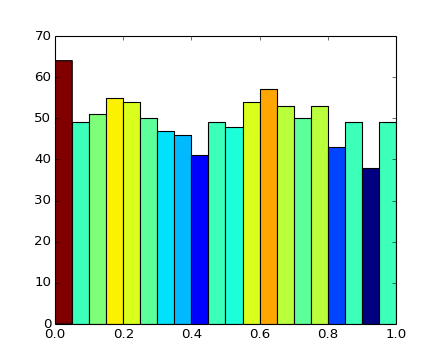# pylab_examples example code: hist_colormapped.py¶```import numpy as np
import matplotlib.pyplot as plt
import matplotlib.cm as cm
import matplotlib.colors as colors

fig, ax = plt.subplots()
Ntotal = 1000
N, bins, patches = ax.hist(np.random.rand(Ntotal), 20)

#I'll color code by height, but you could use any scalar

# we need to normalize the data to 0..1 for the full
# range of the colormap
fracs = N.astype(float)/N.max()
norm = colors.Normalize(fracs.min(), fracs.max())

for thisfrac, thispatch in zip(fracs, patches):
color = cm.jet(norm(thisfrac))
thispatch.set_facecolor(color)

plt.show()
```

Keywords: python, matplotlib, pylab, example, codex (see Search examples)